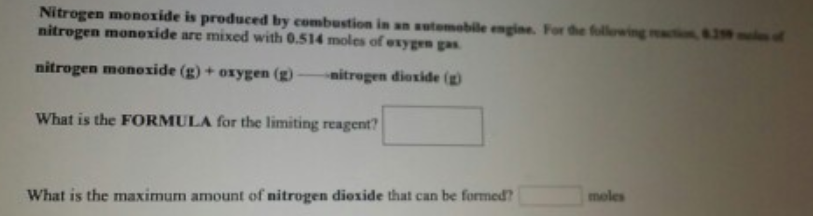# Problem: Nitrogen monoxide is produced by combustion in an automobile engine. For the following reaction, 0.250 moles of nitrogen monoxide are mixed with 0.514 moles of oxygen gas nitrogen monoxide (g) + oxygen (g) → nitrogen dioxide (g) What is the FORMULA for the limiting regent? What is the maximum amount of nitrogen dioxide that can be formed?

###### FREE Expert Solution
90% (114 ratings)###### Problem Details

Nitrogen monoxide is produced by combustion in an automobile engine. For the following reaction, 0.250 moles of nitrogen monoxide are mixed with 0.514 moles of oxygen gas

nitrogen monoxide (g) + oxygen (g) → nitrogen dioxide (g)

What is the FORMULA for the limiting regent?

What is the maximum amount of nitrogen dioxide that can be formed?